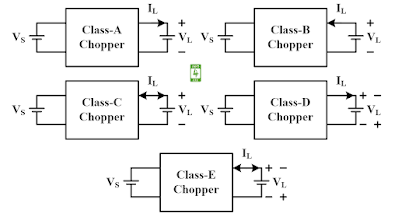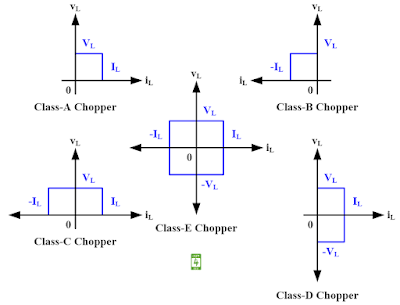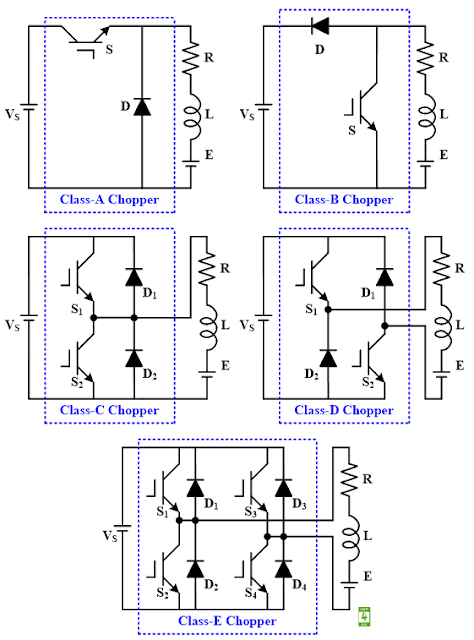Choppers are of five types i.e. Class-A, Class-B, Class-C, Class-D, and Class-E. These choppers can be classified in three ways i.e. based on load voltage and current, based on quadrant operation, and based on the circuit diagram.

### Classification of Chopper based on Load Voltage and Current

The classification of the chopper based on load voltage and current is given in Fig. 1.
1. In the class-A chopper, the load voltage and current both are positive.
2. In the class-B chopper, the load voltage is positive, and the current is negative.
3. In the class-C chopper, the load voltage is positive, and the current may be either positive or negative.
4. In the class-D chopper, the load voltage is either positive or negative, and the current is positive.
5. In the class-E chopper, the load voltage is either positive or negative, and the current is also either positive or negative.Fig. 1 Classification of chopper based on load voltage and current

### Classification of Chopper based on Quadrant Operation

The classification of the chopper based on quadrant operation is given in Fig. 2.
1. Class-A chopper is a single quadrant chopper and lies in the first quadrant.
2. Class-B chopper is a single quadrant chopper and lies in the second quadrant.
3. Class-C chopper is a two-quadrant chopper and lies in the first and second quadrants.
4. Class-D chopper is a two-quadrant chopper and lies in the first and fourth quadrants.
5. Class-E chopper is a four-quadrant chopper and lies in all four quadrants.Fig. 2 Classification of chopper based on quadrant operation

### Classification of Chopper based on Circuit Diagram

The classification of the chopper based on the circuit diagram is given in Fig. 3.
1. Class-A and Class-B chopper consists of one IGBT switch and one diode.
2. Class-C and Class-D chopper consists of two IGBT switches and two diodes.
3. Class-E chopper consists of four IGBT switches and four diodes.Fig. 3 Classification of Chopper based on Circuit Diagram

#### Reference

M. H. Rashid, “Power Electronics: Circuits, Devices, and Applications,” Prentice Hall India, Second Edition, pp. 312-316, 2006.